# History of Trigonometry - Part 2

##### Age 11 to 18

Published 2010 Revised 2022

## Early Astronomy and the Beginnings of a Mathematical Science

This is the second of three articles on the History of Trigonometry. Part 1 can be found here and part 3 can be found here.

### 5. The Beginnings of Mathematical Astronomy

As we have seen, the Babylonians were interested in predicting the time at which a particular celestial event would occur whereas Greek astronomy became much more interested in predicting where a celestial body would be at any particular time. This contrast is clearly illustrated by the development of a geometrical model of the motions of the heavens by the Greek mathematician Eudoxus.

#### Eudoxus (408-355 BCE)

The original belief of an Earth-centred system in perfect circles came from the Pythagoreans (6th/5th centuries BCE) and was discussed and elaborated over the years. Eudoxus was taught by Archytas, one of the leading Pythagorean philosophers of his time, who maintained that the most perfect shape was a circle, so Eudoxus proposed a system of concentric spheres to describe the movement of the planets. This idea, where the planets moved about the Earth in circles on the surfaces of different spheres, remained the widely held theory of the universe until it was challenged by Nicolaus Copernicus in 1543. The simple idea is shown in the diagram below. However, there were enough data from the Babylonians and others to show that the system was much more complicated, and Eudoxus finally produced a system with 27 spheres altogether.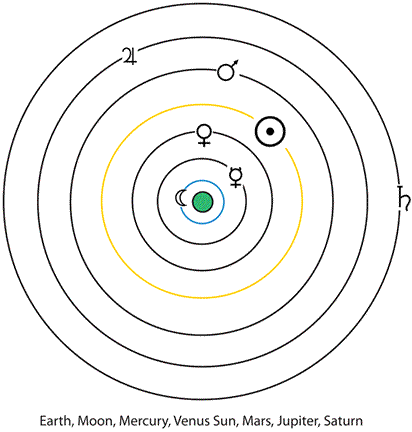Using data derived from the Babylonian astronomers, Eudoxus was able to calculate that the variation in latitude of the Moon was about $+/- 5^\circ$ (in our terms), and the angle between the equator and the ecliptic [See Note 1 below] was measured as $\frac{1}{15}$ of a circle or $24^\circ$ which is the angle subtended at the centre of a 15 sided polygon. This construction was included in Euclid's Elements Book IV proposition 16, because it was so useful for astronomy.

Eudoxus also developed the theory of proportion, now contained in Book V of Euclid's Elements, which became the major logical tool for empirical investigaton until the seventeenth century, and his method of exhaustion became the foundation of the technique developed by Archimedes (287-212 BCE) for finding areas of circles and other shapes.

The theory of the solar system that the Greeks developed was principally an "explanatory device" more descriptive than predictive, and as observations became more accurate, it needed constant revision and modification.

#### Aristarchus (310-230 BCE)

Using Eudoxus' theory of proportion, Aristarchus measured the relative sizes and distances to the Moon and Sun and found the Sun to be bigger than Earth! So, he reasoned that the Sun rather than Earth is the centre of the Universe and the Earth is one of the planets.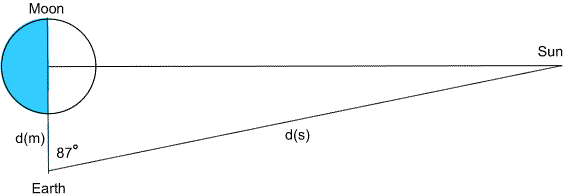In his book On the Sizes and Distances of the Sun and the Moon he assumed that the Earth is at the centre of a sphere on which the Moon moves, and at half Moon the edge of the shadow is in a direct line with the eye. At this time he claimed that its angular distance from the Sun is $87^\circ$ (actually it is about $89^\circ$). Using the time taken for a complete eclipse he estimated that the width of the Earth's shadow is two Moon diameters (actually it is nearer three), and the Moon had an angular diameter of $2^\circ$ (this is about four times too large).

Using these hypotheses he obtained the ratio of the distance from the earth to the sun as:

$$18 \leq \frac{d_1}{d_m} \leq 20$$

Today, this would simply involve finding $cos 87^\circ ( = sin 3^\circ)$ but the concepts of $cos$ and $sin$ were not thought of until much later.

Applying his logical reasoning to the theory of proportion, he arrived at an estimate for the distance to the Sun of about 19 times the distance to the Moon. This estimate was generally accepted for the next 2,000 years.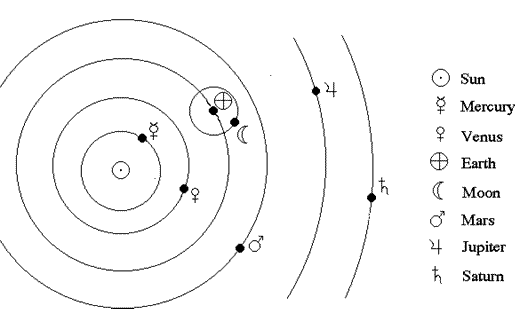Aristarchus' observations showed that Eudoxus' model was not able to account for some of the simplest motions of the planets, and so Greek mathematicians like Apollonius (262-190 BCE) managed to find more complicated geometrical constructions in order to keep the Earth at the centre of the universe.

Although it might seem like a missed opportunity to us for Greek scholars to abandon Aristarchus' model of the solar system, even though he had shown that the Sun was larger than the Earth, there was no real evidence to show that the Sun was the centre of our system. The fact that the Sun might be larger than the Earth was no reason to suppose that the Sun was at the centre when the obvious apparent rotation of the Sun, Moon and Planets around the Earth was so strong.

#### Hipparchus (190-120 BCE)

The first known table of chords was produced by the Greek mathematician Hipparchus in about 140 BC. Although nothing has survived, it is claimed that he wrote twelve books of tables of chords, but Neugebauer shows this to be impossible. Hipparchus developed great observational skills, improved the design of instruments and compiled a catalogue of about 850 stars. He also tried to improve the geometrical model of the universe. His most important discovery was the "Precession of the Equinoxes". Since the Earth's axis is tilted with respect to the stars it slowly describes a circle. This results in the intersection points of the celestial equator and the ecliptic (the equinoxes) changing slowly. Precession also explains why the "Polar Star" changes its position in the heavens over the centuries. [See details on precession here]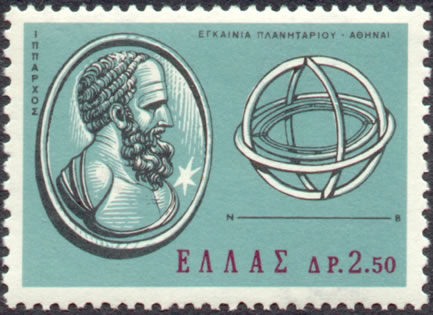Hipparchus is credited with constructing the first known table of chords. The importance of Hipparchus' achievement in doing this was to change the mathematical tools involved from the arithmetical 'procedures' of the Babylonian scholars to a geometrical device' , namely the use of arcs of a circle imagined to be on the surface of the 'heavenly sphere'. Even so, he was still using the new technique to investigate the location of heavenly bodies, and the process was still clearly embedded in astronomy. Trigonometry, as a separately identifiable science in its own right, does not appear before the Arab scholars developed it much further in the eleventh and twelfth centuries CE.[See Note 2 below]

#### Menelaos (70-130 BCE)

In about 100 BCE Menelaos compiled a Book of Spherical Proportions Sphaerica, in which he set up the basis for treating spherical triangles by using arcs of great circles instead of arcs of parallel circles on the sphere. [To learn more about spherical triangles go here].

The plane triangle version of the theorem states:

If a straight line crosses the three sides of a triangle (one of the sides has to be produced) then the product of three of the non-adjacent segments thus formed is equal to the product of the other three segments of the triangle.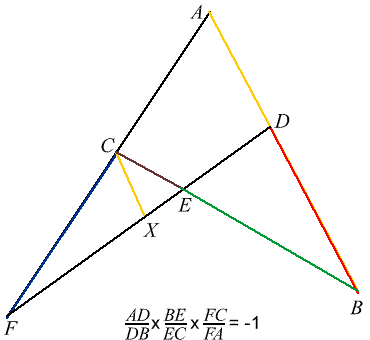This proof uses similar triangles

$ABC$ is a triangle with a transversal $DE$ that cuts $AC$ produced in$F$

First, insert a construction line $CX$ parallel to $AD$

($CX$ will be eliminated later). Now triangles $ADF$ and $CXF$ are similar, so $$\frac{AD}{CX}=\frac{FA}{FC} \mbox{ , and in the cyclic quadrilateral, }BFCD, \frac{BE}{EC}=\frac{DB}{CX}$$
$$\mbox{Multiply }\frac{AD}{CX}=\frac{FA}{FC} \mbox{ by equal quantities,}$$
$$\mbox{and } \frac{AD}{CX}\times\frac{BE}{EC}= \frac{FA}{FC} \times\frac{DB}{CX}$$
$$\mbox{Insert the ratio } \frac{CX}{CX} (=1) \mbox{ into the Left Hand Side product before cancellation, and}$$
$$\frac{AD}{DB}\times\frac{BE}{EC}\times\frac{FC}{FA}=1$$
Note: The orientation of $FC$ is important.

Menelaus produced a spherical triangle version of this theorem and a modification of it appears in Ptolemy's Almagest. [This is explored in the NRICH problem Pythagoras on a Sphere]

#### Claudius Ptolemy (c85-c165 CE)

Ptolemy was the most influential Greek astronomer of this time. He supported the geocentric theory of the universe, and his book The Mathematical Compilation dominated astronomical thought until Copernicus (1473-1543) published his heliocentric theory in 1543.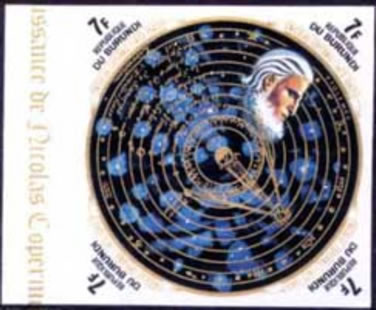The aim of the Almagest was to provide numerical data for astronomical phenomena and observation and to explain the empirical foundations and theoretical reasons for the data.

The Almagest contains a collection of all the then known astronomical knowledge: geometrical and numerical procedures, the longitude and latitude of heavenly bodies, information about parallax, the distance and relative sizes of the Sun and the Moon, lunar theory, solar motion, and the occurrence of eclipses, transits and occultations. This was the reason the Arab scholars called it "Al Megiste" (the great one) and the name has remained.

Ptolemy was the author of a new table of chords, based on exactly the same principles inherited from the Babylonians, and using more sophisticated observational techniques, more accurate data, and the new mathematics of Euclid, Apollonius Archimedes and Menelaos.

He divided the circle into 360 parts and used a diameter of 120 units, and calculated in the traditional sexagesimal numbers that astronomers had been using for two thousand years. Ptolemy calculated chords by constructing a series of regular polygons in a circle as shown by Euclid.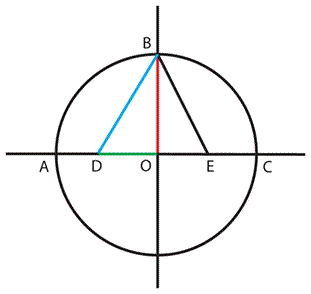The Chords of regular polygons of 3, 4, 5, 6 and 10 sides respectively subtend angles of $120^\circ, 90^\circ, 72^\circ, 60^\circ$, and $10^\circ$ at the centre of a circle. By continued bisection and interpolaton he could find chords for many other angles which he expressed as ratios of chord to diameter finally arriving at chords of angles of $\frac{1}{2}^\circ$ where in our terms, chord $\mathbf{\alpha = 120 \mbox{ }Sin \alpha}$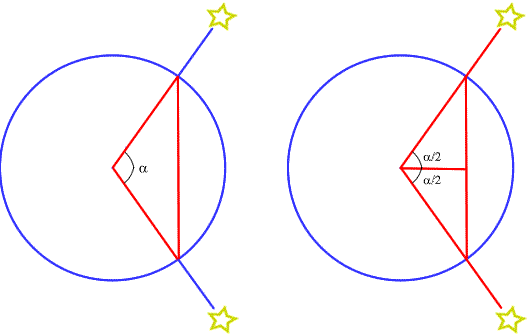In sexagesimal notation, the chords for the regular polygons are:

chord $36^\circ$ = 37; 4, 55,
chord $60^\circ$ = 1, 0; 0
chord $72^\circ$ = 1, 10; 32. 3
chord $90^\circ$ = 1, 24; 51, 10

from Pythagoras' theorem:
chord $^2 \alpha$ + chord$^2 (180 -\alpha ) = 120^2$
he obtained chord $120^\circ$ = 1, 43; 55, 23. and chord $144^\circ$ = 1, 54; 7, 37.

Converting Ptolemy's chord calculations with base ten:

for chord $60^2$ the sexagesimal 1, 0; 0. is 60 in base ten and 120 sin $30^\circ$ = 120 $\times \frac{1}{2}$ or 60 units, which is the radius of the circle.
similarly, chord $90^\circ$ = 1, 24; 51, 10. or in base ten,
chord $90^\circ$ = 60 + 24 + $\frac{3060 + 10}{3600}$ which is 84.8527 units
and chord $90^2$ = 120 x sin 450 or 120 x or 84.8536 units

Using what is now called "Ptolemy's theorem" (this property of cyclic quadrilaterals was known much earlier) he set up a system where one of the sides of the quadrilateral was a diameter, that enabled him to calculate sums and products of chords.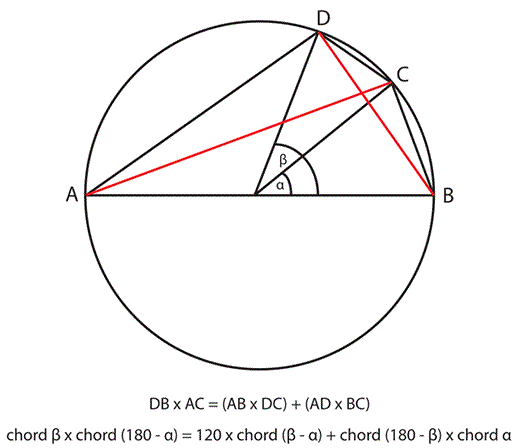Converting the chords expression to modern notation, and putting $x = \frac{\beta}{2}$ and $y = \frac{\alpha}{2}$ this is equivalent to:
$$sin (x - y) = sin x cos y - cos x sin y$$

Similarly he obtained
$$sin (x + y) = sin x cos y + cos x sin y$$
and also derived
$$2 sin^2 x = 1 - cos 2x$$

Using the table of chords with the geometry and formulae above, Ptolemy was able to solve all the triangles he needed to create his table of chords in steps of half degrees from $\frac{1}{2} \circ$ to $180 ^\circ$ which is equivalent to a table of sines from $\frac{1}{4} ^\circ$ to $90 ^\circ$.

### 6. Ptolemy's use of Spherical Triangles

Menlaos' Sphaerica was the major work that established the science of spherical geometry, and this was, in a sense, a non-Euclidean geometry where the sides of a triangle were formed by the intersections of three great circles on a sphere, and the angle sum of a triangle could be more than $180^0$. At the time, it was not seen as a radically new geometry, but more as an extension of Euclid, and most of the time the triangles used were constructed with only one right angle. At this time, spherical geometry was included in the quadrivum (arithmetic, geometry, music, astronomy), and taught as part of astronomy. The new relationships developed were possible by the use of proportional reasoning, a direct result of Eudoxus' theory. Ptolemy was well aware of the new possibilities, because finding the distance between two stars was equivalent to measuring an arc of a circle, and he adapted the spherical geometry for use with tables of chords.

### 7. Passing on the Knowledge

By this time, mathematicians and astronomers had developed a complex mathematically based science, had a wide range of geometrical techniques whereby they measured the Earth, estimated the distances of the Moon and the Sun, developed a theory of the movement of the planets, and precisely catalogued hundreds of stars. A substantial body of geometrically based mathematics had been developed and scholars made commentaries on the works of Euclid, Apollonius, Archimedes, and others. In the next centuries, Diophantos wrote his Arithmetica, which was to inspire Fermat centuries later, Pappus recorded much of the earlier learning for later generations, and contacts along the trade routes began to be made with people in India and China. In September 622 Mohammed made his famous escape from persecution in Mecca to safety in Medina, and within two hundred years, the Arab culture had established an empire from India through the Middle East and North Africa and into Spain.

Pedagogical notes to support this article can be found in the Teachers' Notes accompanying this resource.

### Part 3 of the History of Trigonometry:

The next part of the story can be found here. It tells how from the 10th century CE Arab scholars collected all kinds of knowledge from 'even as far as China'. They consolidated the techniques of astronomy, and established trigonometry as a distinct branch of mathematics. During the 12th and 13th centuries many of the Arab works were translated into Latin and the ideas passed into the hands of European scholars. Further contributions were made and new material added, and by 1464, when Regiomontanus had read the available material in Greek and Latin, he completed his major work, On Triangles of Every Kind where trigonometry was set out as a series of logically connected theorems, in the style of Euclid.

### References

Katz, V. (1998) A History of Mathematics. New York. Addison Wesley. Recommended as the best general history of mathematics currently available. There is good coverage of aspects of astronomy in antiquity, and the discussion on 'functions' (p. 156) is worth reading. Trigonometry is dealt with in sections on Ancient Civilisations, Mediaeval Europe, Renaissance Europe

Katz, V. (Ed.) (2007) The Mathematics of Egypt, Mesopotamia, China, India, and Islam. Princeton. Princeton University Press. This book contains a wealth of up-to-date information on mathematics and some aspects of astronomy in these ancient civilisations.

Linton, C. M. (2004) From Eudoxus to Einstein: A History of Mathematical Astronomy. Cambridge University Press The first chapter deals with ancient people and early Greek astronomy.

Needham, J. (1959) Science and Civilisation in China. Vol. 3. Mathematics and the Sciences of the Heavens and the Earth. Cambridge University Press.

Neugebauer, O. (1983)(1955) Astronomical Cuneiform Texts. Vol. 1 The Moon. Heidelberg. Springer-Verlag These two books are the big classics on China and Mesopotamia, but much work has been done in these areas since the 1950s.

Neugebauer, O. (1969) (original 1952) The Exact Sciences in Antiquity. New York. Dover Books. Still available, this is a more popular book and contains much information on Egypt, Babylon and Greek Science.

Plofker, K. (2009) Mathematics in India. Princeton. Princeton University Press. This is the most recent book on the history of Mathematics in India by a renowned expert.

This Wikipedia site on the Celestial Spheres provides much information on the change from the Ptolemaic to the Copernican system:

http://en.wikipedia.org/wiki/Celestial_spheres

Menalaos' Theorem: a neat proof step by step is here: